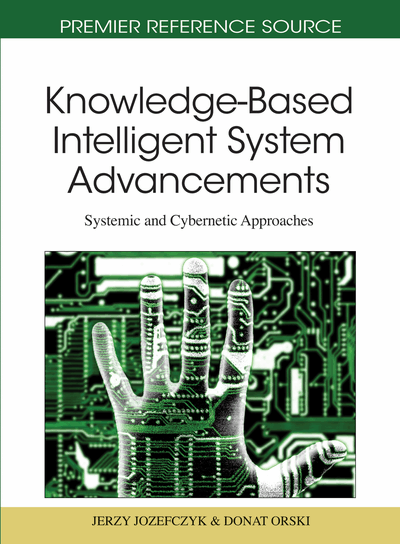# Object Recognition via Contour Points Reconstruction Using Hurwitz - Radon Matrices

Dariusz Jakóbczak (Technical University of Koszalin, Poland)
DOI: 10.4018/978-1-61692-811-7.ch005
Available
\$29.50
No Current Special Offers

## Abstract

Object recognition is one of the topics of artificial intelligence, computer vision, image processing and machine vision. The classical problem in these areas of computer science is that of determining object via characteristic features. Important feature of the object is its contour. Accurate reconstruction of contour points leads to possibility to compare the unknown object with models of specified objects. The key information about the object is the set of contour points which are treated as interpolation nodes. Classical interpolations (Lagrange or Newton polynomials) are useless for precise reconstruction of the contour. The chapter is dealing with proposed method of contour reconstruction via curves interpolation. First stage consists in computing the contour points of the object to be recognized. Then one can compare models of known objects, given by the sets of contour points, with coordinates of interpolated points of unknown object. Contour points reconstruction and curve interpolation is possible using new method of Hurwitz - Radon Matrices.
Chapter Preview
Top

## Background

The following question is important in mathematics and computer sciences: is it possible to find a method of curve interpolation in the plane without building the interpolation polynomials? This chapter aims at giving the positive answer to this question. Current methods of curve interpolation based on classical polynomial interpolation: Newton, Lagrange or Hermite polynomials and spline curves which are piecewise polynomials (Dahlquist & Bjoerck, 1974). Classical methods are useless to interpolate the function that fails to be differentiable at one point, for example the absolute value function f(x) = |x|at x = 0. If point (0;0) is one of the interpolation nodes, then precise polynomial interpolation of the absolute value function is impossible. Also when the graph of interpolated function differs from the shape of polynomials considerably, for example f(x) = 1/x, interpolation is very hard because of existing local extrema of polynomial. We cannot forget about the Runge’s phenomenon: when interpolation nodes are equidistance then high-order polynomial oscillates toward the end of the interval, for example close to -1 and 1 with function f(x) = 1/(1+25x2) (Ralston, 1965). MHR method is free of these bad examples. Computational algorithm is considered and then we have to talk about time. Complexity of calculations for one unknown point in Lagrange or Newton interpolation based on n nodes is connected with the computational cost of rank O(n2).

## Complete Chapter List

Search this Book:
Reset